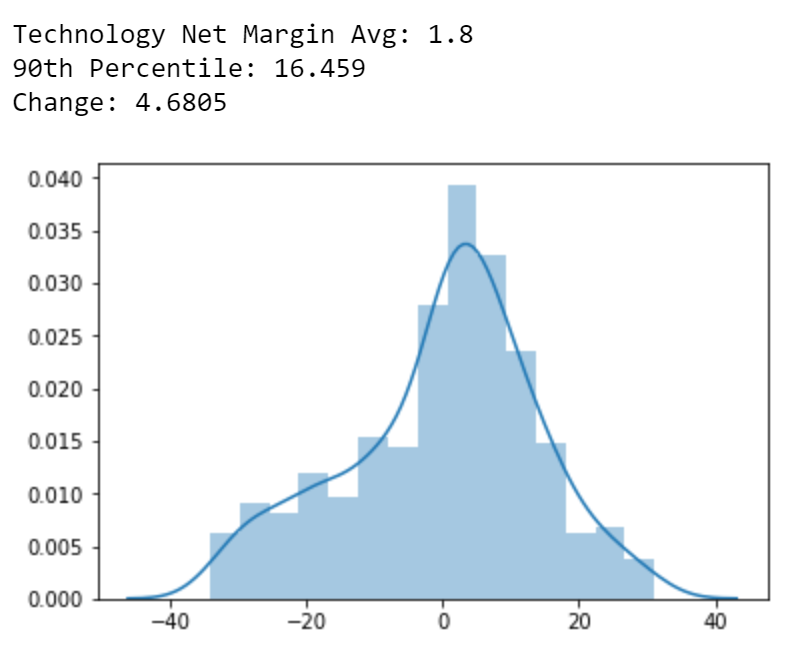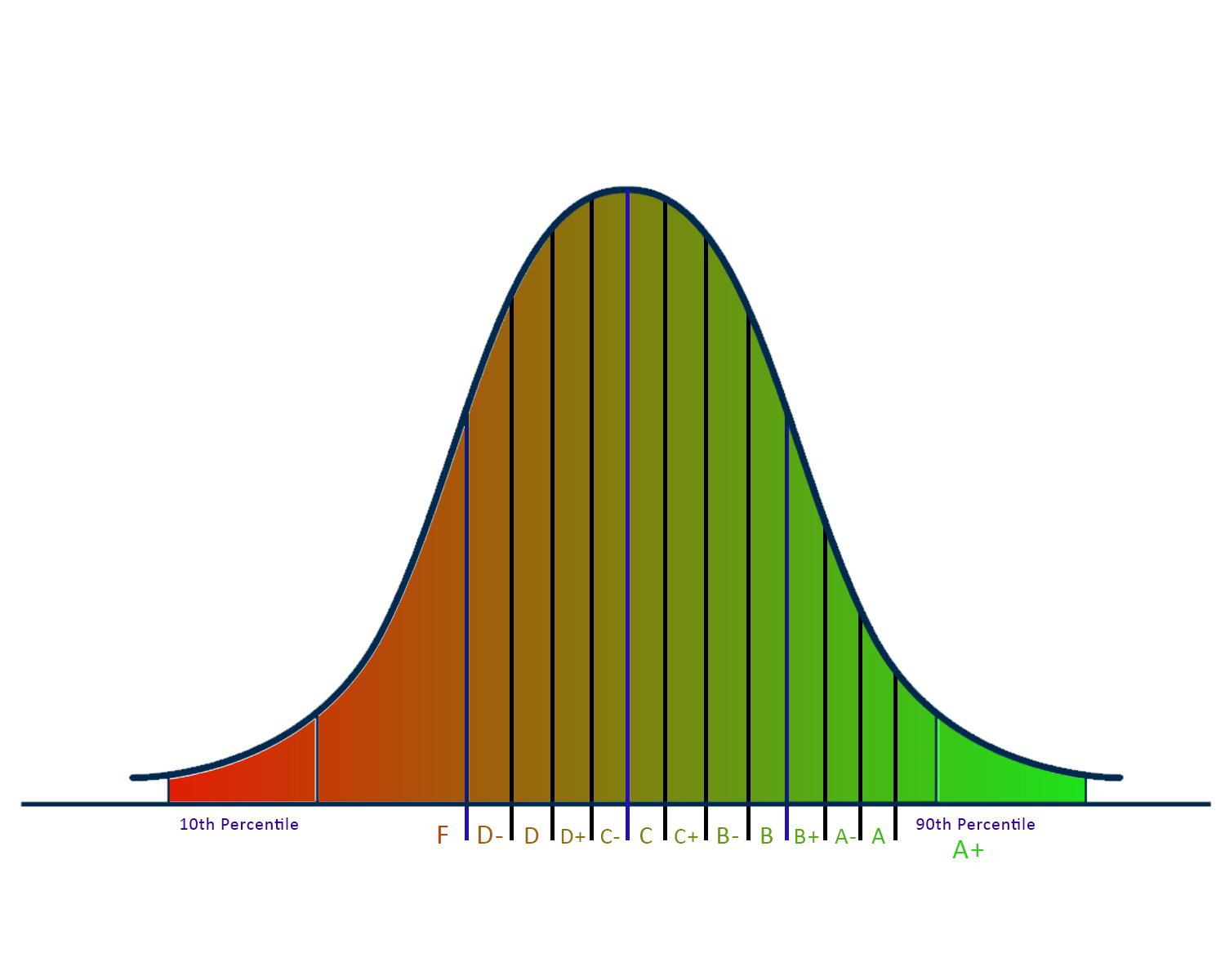## Automated-Fundamental-Analysis

Python program that rates stocks out of 100 based on valuation, profitability, growth, and price performance metrics, relative to the company's sector.

The grading system used in this program is based on the normal distribution of values for a certain metric for a specified sector. For example, if I want to grade the Net Margin of a stock in the Technology sector, I look at the net margins of all the stocks in the technology sector and grade the stock's net margin based on its percentile in the distribution of values.

In the figure below, we see that the average net margin for a stock in technology sector is 1.8% and the 90th percentile is 16.45%. The grading system utilized in the program takes the standard deviation of the set of values after removing outliers and divides that number by 3, which is represented by the 'Change' value shown in the figure, equaling 4.68. This is the value that is used to grade each metric for each stock.The figure shown below represents exactly how each metric is graded, where each bar in the graph is representative of 1 increment of the 'Change' value.
Based on this figure and the 'Change' value, a stock in the technology sector with a net margin of:

• 17% is rated A+
• 15% is rated A
• 11% is rated A-After all the metrics in each category of valuation, profitability, growth, and price performance are graded, the grades are then converted to numbers and then the average of the values is computed. To get the overall rating of a stock, these numerical ratings for each category are added together and multiplied to get a score out of 100.

# How to Run the Code

1. Clone the repository `git clone https://github.com/faizancodes/Automated-Fundamental-Analysis.git`
2. Run `cd Automated-Fundamental-Analysis`
3. Download all the dependencies `pip install -r requirements.txt`
4. Run `getsectordata.py`, this file generates necessary data for each sector in the stock market
5. Run `stockratings.py`, this will generate the csv files you can use for fundamental analysis
6. Open the csv files in the StockRatings folder created from the program through excel and analyze!